向量点积的几点应用 - WebGL

-- wikipedia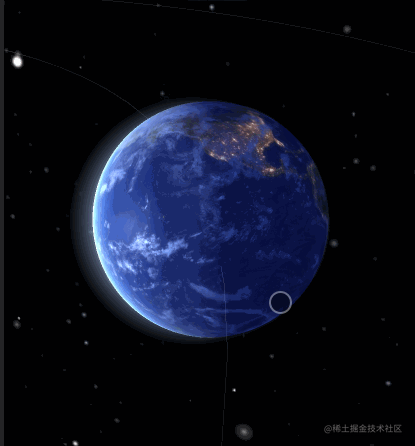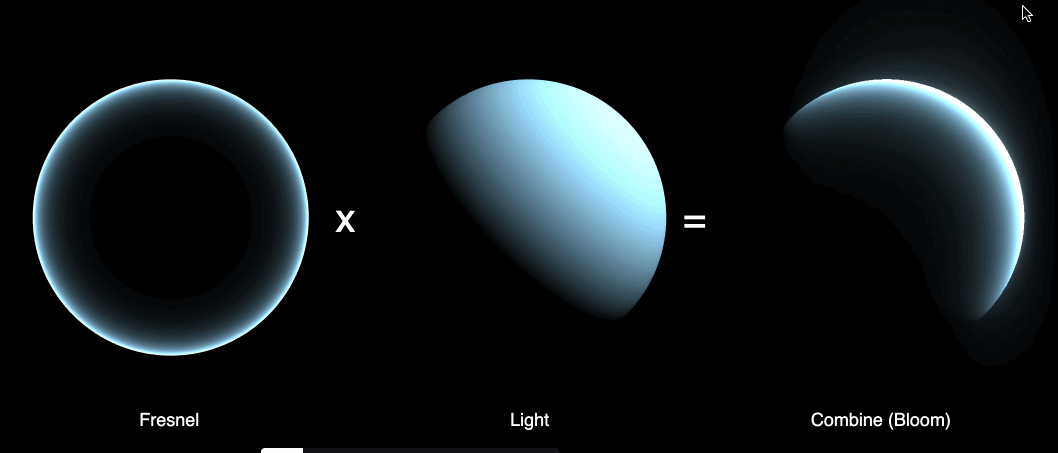// 顶点着色器
uniform vec3 viewVector;
varying vec3 vNormal;
varying float intensity;
uniform float uAperture;
uniform float uPower;

void main() {
vNormal = normalize( normalMatrix * normal );
vec3 viewNormal = normalize( normalMatrix * viewVector );
intensity = pow(uAperture - dot(vNormal, viewNormal), uPower );
gl_Position = projectionMatrix * modelViewMatrix * vec4( position, 1.0 );
}

// 片元着色器
uniform vec4 uColor;
varying vec3 vNormal;
varying float intensity;
uniform vec3 uLightDir;

void main() {
vec4 lDirection = viewMatrix * vec4( uLightDir, 0.0 );
vec3 dirVector = normalize( lDirection.xyz );
float lightShine = max( 1.5 * dot( vNormal, dirVector ), 0.0 );
gl_FragColor = intensity * vec4( vec3(lightShine)*uColor.xyz, 1.0 );
}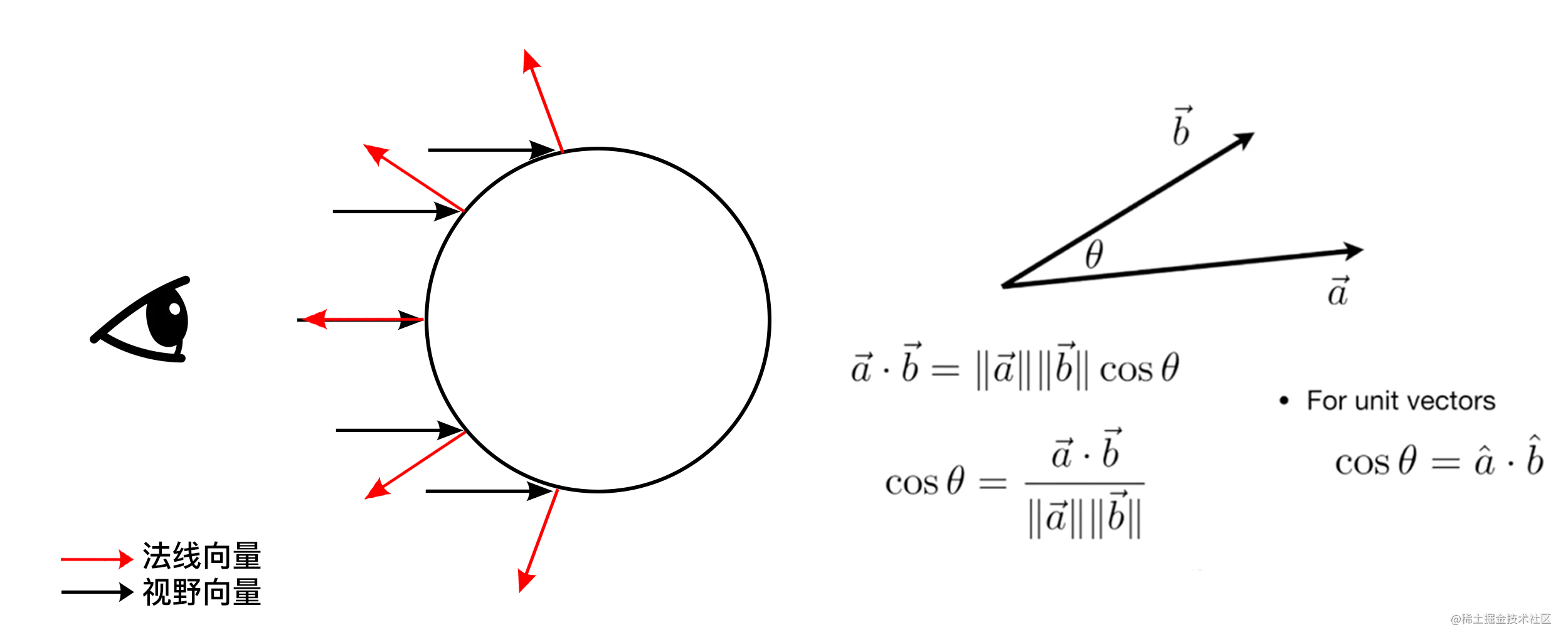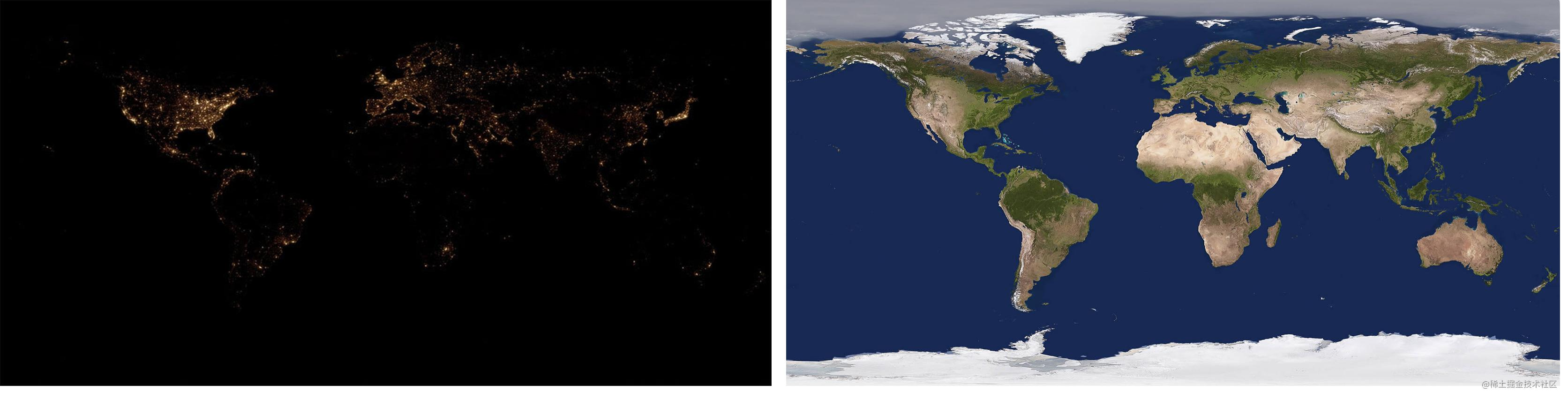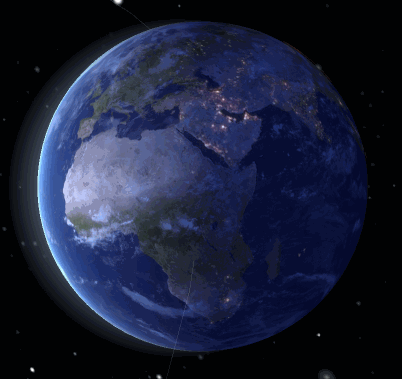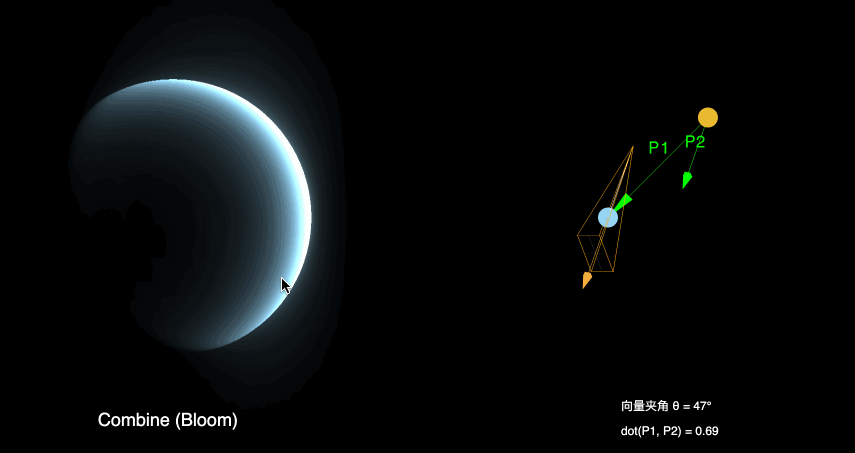应用二：屏蔽视野遮挡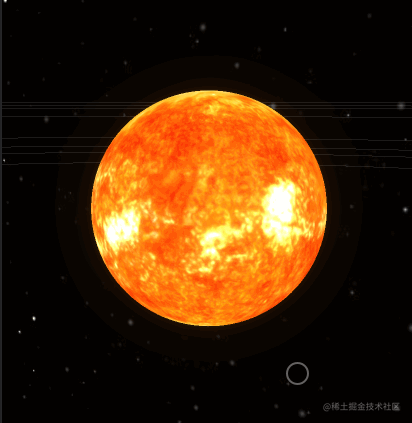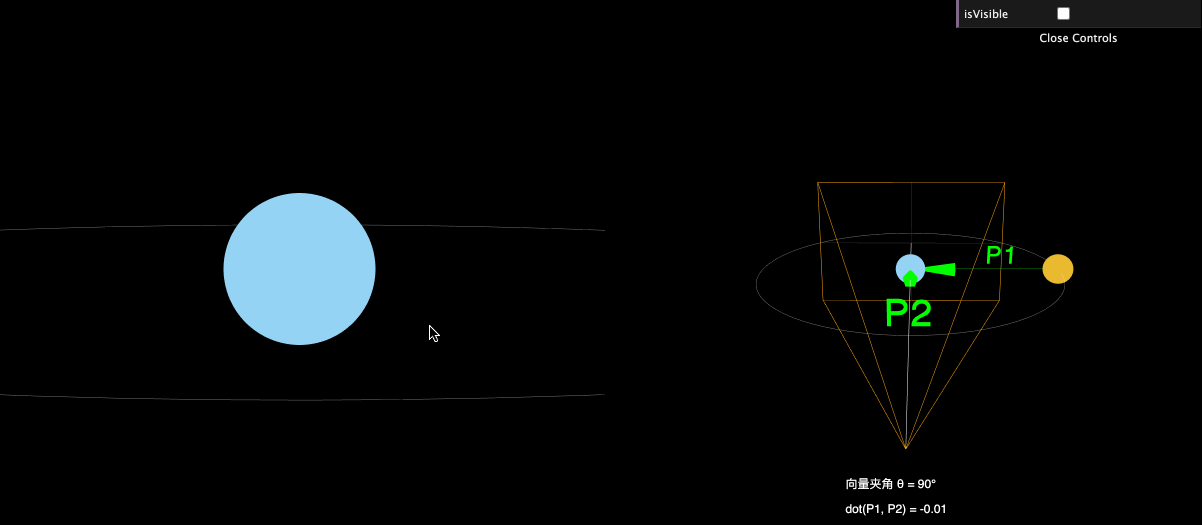应用三：约束摄像机运动幅度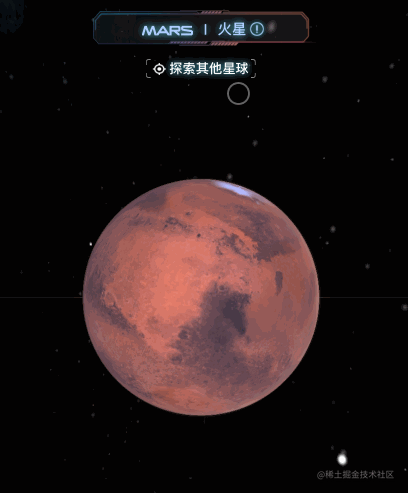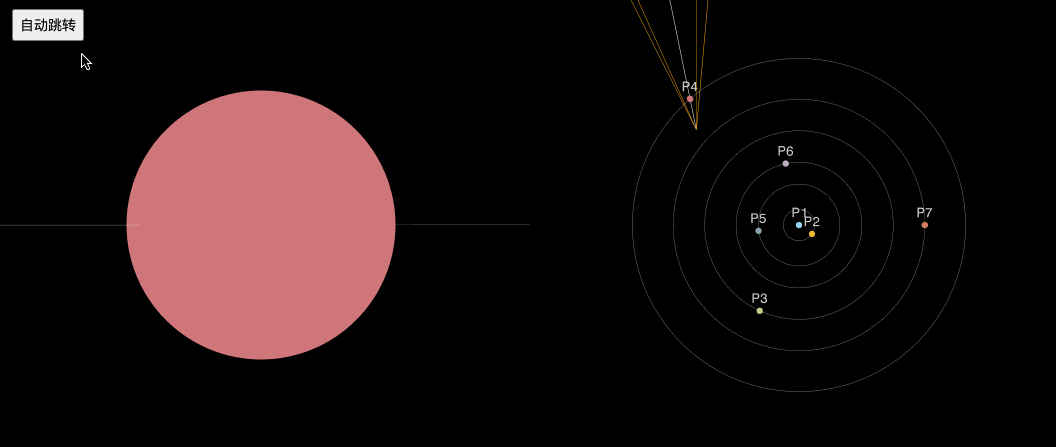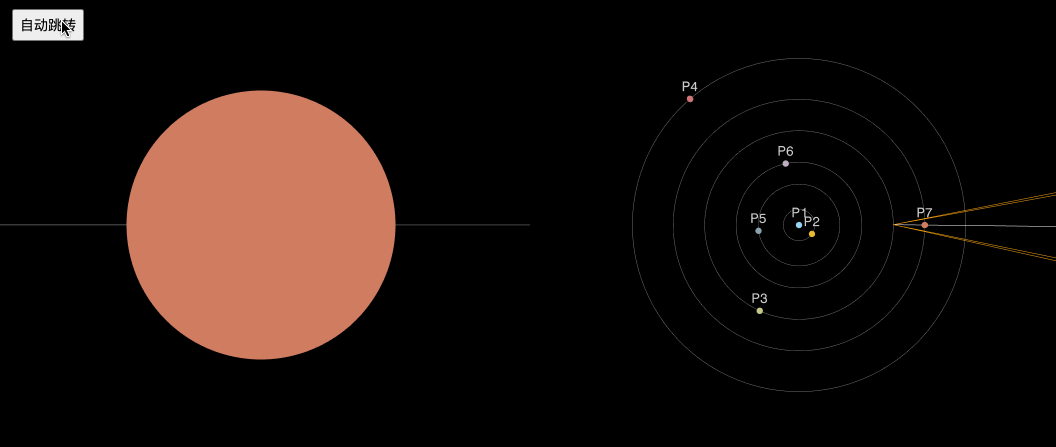H5 体验二维码：